## Alternative Formulation of the Second Law of Thermodynamics

The thermodynamic state of a substance is defined by the quantities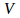– volume,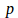– pressure,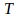– Temperature and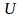– internal energy.

These four quanties are related by the equations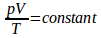and one equation for the internal energy eg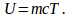This means we can choose any two of these quantities as independent andf express the other two – dependent – variables in terms of the independent variables.

The second law of thermodynamics can be written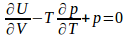(1) whereandare independent.

Or as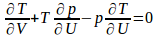whereandare independent (2)

Or as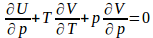whereandare independent (3)

Or as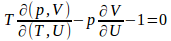whereandare independent (4)

Or as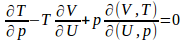where U and p are independent (5)

Or as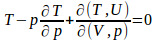whereandare independent. (6)

To show (1) write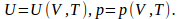Then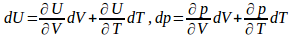(7)

Now let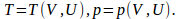Then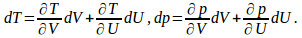(8)

Hence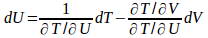and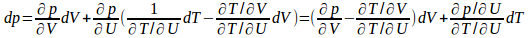Equate this expression with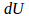and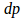from (7) to give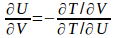and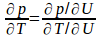Hence we can write (1) as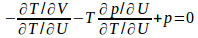or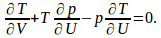he proof for (3) is similar.

To prove (4) takeandas independent, then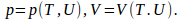Then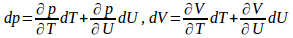Rearrange the second of these to give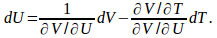Substitute into the first to give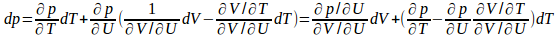Compare these two equations with equations (7) to give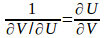and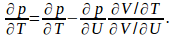Substitute these into (1) to obtain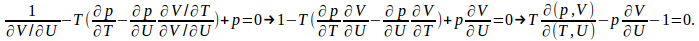(5) and (6) can be similarly proved.

#### Add commentRefresh